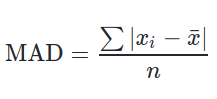# Python | Pandas Series.mad() to calculate Mean Absolute Deviation of a Series

Pandas provide a method to make Calculation of MAD (Mean Absolute Deviation) very easy. MAD is defined as average distance between each value and mean.

The formula used to calculate MAD is:Parameters:
axis: 0 or ‘index’ for row wise operation and 1 or ‘columns’ for column wise operation.
skipna: Includes NaN values too if False, Result will also be NaN even if a single Null value is included.
level: Defines level name or number in case of multilevel series.

Return Type: Float value

Example #1:
In this example, a Series is created from a Python List using Pandas .Series() method. The .mad() method is called on series with all default parameters.

 `# importing pandas module  ` `import` `pandas as pd  ` `   `  `# importing numpy module  ` `import` `numpy as np  ` `   `  `# creating list ` `list` `=``[``5``, ``12``, ``1``, ``0``, ``4``, ``22``, ``15``, ``3``, ``9``] ` ` `  `# creating series ` `series ``=` `pd.Series(``list``) ` ` `  `# calling .mad() method ` `result ``=` `series.mad() ` ` `  `# display ` `result `

Output:

`5.876543209876543`

Explanation:

Calculating Mean of series mean = (5+12+1+0+4+22+15+3+9) / 9 = 7.8888

MAD = | (5-7.88)+(12-7.88)+(1-7.88)+(0-7.88)+(4-7.88)+(22-7.88)+(15-7.88)+(3-7.88)+(9-7.88)) | / 9.00

MAD = (2.88 + 4.12 + 6.88 + 7.88 + 3.88 + 14.12 + 7.12 + 4.88 + 1.12) / 9.00

MAD = 5.8755 (More accurately = 5.876543209876543)

My Personal Notes arrow_drop_upCheck out this Author's contributed articles.

If you like GeeksforGeeks and would like to contribute, you can also write an article using contribute.geeksforgeeks.org or mail your article to contribute@geeksforgeeks.org. See your article appearing on the GeeksforGeeks main page and help other Geeks.

Please Improve this article if you find anything incorrect by clicking on the "Improve Article" button below.

Improved By : Akanksha_Rai

Article Tags :

Be the First to upvote.

Please write to us at contribute@geeksforgeeks.org to report any issue with the above content.